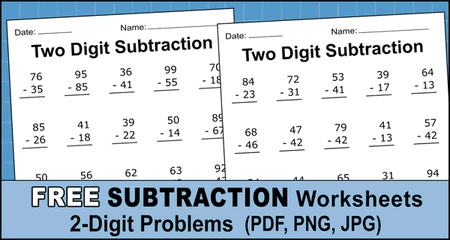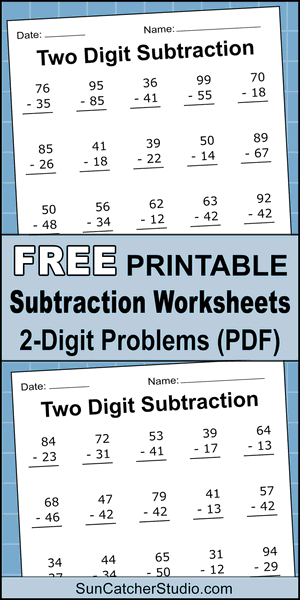## Two-Digit Subtraction Worksheets (Printable Math Drills)

Free printable two digit subtraction worksheets (2-digit math problems) in PDF format. Use these worksheets to help your child or students learn math. For more ideas see math drills and one digit subtraction problems and random math problems generator.Download your free printable subtraction worksheets by selecting either “PDF format” or “PNG format”. You can also change the colors by selecting “Edit / Save”. See notes below.

## Free Printable Two-Digit Subtraction Worksheets (PDF)

• All 2-digit subtraction worksheets and answers in one document.
• PDF format

NOTE: You can specify new colors for your subtraction worksheets (subtraction problems) by pressing the “Edit / Save” button. After selecting new colors, specify the height as “3000 pixels” to ensure high resolution and to make sure your math problems fit on the entire piece of paper.

Subtraction worksheets and educational subtraction math problems can be invaluable tools when kids are learning about math and subtracting numbers. Use these free two digit subtraction math drills at home or at school. Problems include both 2-digit subtraction with regrouping and two digit subtraction without regrouping. Simply click on the worksheet you want, then download and print. These 2-digit subtraction worksheets can make learning math fun. Great for kindergarten, 1st grade, 2nd grade, 3rd grade, and 4th grade classes.

## More FREE Printable Paper, Math Charts, Worksheets, etc.

Find free award winning printable paper, worksheets, and designs to help you and your kids succeed. Discover free graph paper and coordinate grid paper. Find a big selection of printable lined paper including wide ruled, college ruled, narrow ruled, handwriting paper, and kindergarten paper. Stay organized at school, home, and work with cute weekly planners and daily planners and printable calendars. Or keep organized with a To-do list a grocery list or a meal planner.

Discover a big selection of math drills, charts, and worksheets. In particular, you will find multiplication charts, division charts, subtraction tables, and addition charts along with math worksheets and math drills. Also find word search puzzles and a word search generator.
Create stunning personalized gifts or a visualization of your data using the Free word cloud generator.
All paper designs and worksheets can be printed or downloaded in PNG, JPG, PDF, or SVG (Scalable Vector Graphics) format.

Pin for later!